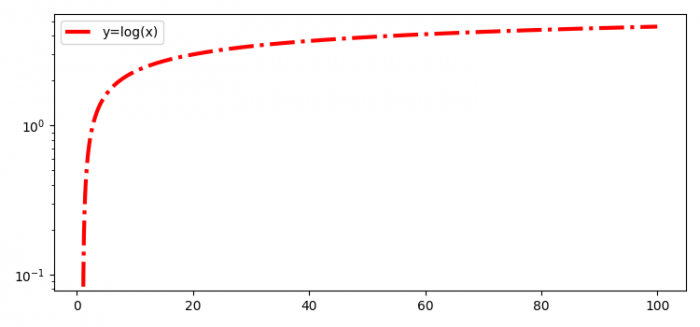# How to visualize values on logarithmic scale on matplotalib?

MatplotlibPythonData Visualization

#### Python Data Science basics with Numpy, Pandas and Matplotlib

Most Popular

63 Lectures 6 hours

#### Data Visualization using MatPlotLib & Seaborn

11 Lectures 4 hours

#### MatPlotLib with Python

9 Lectures 2.5 hours

To visualize values on logarithmic scale on matplotlib, we can use yscale('log').

## Steps

• Import matplotlib nd numpy.

• Set the figure size and adjust the padding between and around the subplots.

• Create x and y data points using numpy.

• Use yscale('log') to visualize the values on a logarithmic scale.

• Plot x and y data points using plot method.

• Place a legend on the figure.

• To display the figure, use show() method.

## Example

import numpy as np
from matplotlib import pyplot as plt

# Set the figure size
plt.rcParams["figure.figsize"] = [7.50, 3.50]
plt.rcParams["figure.autolayout"] = True

# x and y data points
x = np.linspace(1, 100, 1000)
y = np.log(x)

# logarithmic scale
plt.yscale('log')

# Plot the x and y data points
plt.plot(x, y, c="red", lw=3, linestyle="dashdot", label="y=log(x)")

# Place the legend
plt.legend()

# Display the plot
plt.show()

## Output

It will produce the following output −